# Seed-based connectivity on the surface#

The dataset that is a subset of the enhanced NKI Rockland sample (http://fcon_1000.projects.nitrc.org/indi/enhanced/, Nooner et al, 2012)

Resting state fMRI scans (TR=645ms) of 102 subjects were preprocessed (https://github.com/fliem/nki_nilearn) and projected onto the Freesurfer fsaverage5 template (Dale et al, 1999, Fischl et al, 1999). For this example we use the time series of a single subject’s left hemisphere.

The Destrieux parcellation (Destrieux et al, 2010) in fsaverage5 space as distributed with Freesurfer is used to select a seed region in the posterior cingulate cortex.

Functional connectivity of the seed region to all other cortical nodes in the same hemisphere is calculated using Pearson product-moment correlation coefficient.

The `nilearn.plotting.plot_surf_stat_map` function is used to plot the resulting statistical map on the (inflated) pial surface.

See Plotting brain images for more details on plotting tools.

## Retrieving the data#

```# NKI resting state data from nilearn
from nilearn import datasets

nki_dataset = datasets.fetch_surf_nki_enhanced(n_subjects=1)

# The nki dictionary contains file names for the data
print(('Resting state data of the first subjects on the '
'fsaverag5 surface left hemisphere is at: %s' %
nki_dataset['func_left']))

# Destrieux parcellation for left hemisphere in fsaverage5 space
destrieux_atlas = datasets.fetch_atlas_surf_destrieux()
parcellation = destrieux_atlas['map_left']
labels = destrieux_atlas['labels']

# Fsaverage5 surface template
fsaverage = datasets.fetch_surf_fsaverage()

# The fsaverage dataset contains file names pointing to
# the file locations
print('Fsaverage5 pial surface of left hemisphere is at: '
f"{fsaverage['pial_left']}")
print('Fsaverage5 flatten pial surface of left hemisphere is at: '
f"{fsaverage['flat_left']}")
print('Fsaverage5 inflated surface of left hemisphere is at: '
f"{fsaverage['infl_left']}")
print('Fsaverage5 sulcal depth map of left hemisphere is at: '
f"{fsaverage['sulc_left']}")
print('Fsaverage5 curvature map of left hemisphere is at: '
f"{fsaverage['curv_left']}")
```
```Resting state data of the first subjects on the fsaverag5 surface left hemisphere is at: /home/yasmin/nilearn/nilearn_data/nki_enhanced_surface/A00028185/A00028185_left_preprocessed_fwhm6.gii
Fsaverage5 pial surface of left hemisphere is at: /home/yasmin/nilearn/nilearn/nilearn/datasets/data/fsaverage5/pial_left.gii.gz
Fsaverage5 flatten pial surface of left hemisphere is at: /home/yasmin/nilearn/nilearn/nilearn/datasets/data/fsaverage5/flat_left.gii.gz
Fsaverage5 inflated surface of left hemisphere is at: /home/yasmin/nilearn/nilearn/nilearn/datasets/data/fsaverage5/infl_left.gii.gz
Fsaverage5 sulcal depth map of left hemisphere is at: /home/yasmin/nilearn/nilearn/nilearn/datasets/data/fsaverage5/sulc_left.gii.gz
Fsaverage5 curvature map of left hemisphere is at: /home/yasmin/nilearn/nilearn/nilearn/datasets/data/fsaverage5/curv_left.gii.gz
```

## Extracting the seed time series#

```# Load resting state time series from nilearn
from nilearn import surface

# Extract seed region via label
pcc_region = b'G_cingul-Post-dorsal'

import numpy as np

pcc_labels = np.where(parcellation == labels.index(pcc_region))

# Extract time series from seed region
seed_timeseries = np.mean(timeseries[pcc_labels], axis=0)
```

## Calculating seed-based functional connectivity#

```# Calculate Pearson product-moment correlation coefficient between seed
# time series and timeseries of all cortical nodes of the hemisphere
from scipy import stats

stat_map = np.zeros(timeseries.shape)
for i in range(timeseries.shape):
stat_map[i] = stats.pearsonr(seed_timeseries, timeseries[i])

stat_map[np.where(np.mean(timeseries, axis=1) == 0)] = 0
```
```/home/yasmin/miniconda3/envs/nilearn-dev/lib/python3.10/site-packages/scipy/stats/_stats_py.py:4394: ConstantInputWarning:

An input array is constant; the correlation coefficient is not defined.
```

Display ROI on surface

```# Transform ROI indices in ROI map
pcc_map = np.zeros(parcellation.shape, dtype=int)
pcc_map[pcc_labels] = 1

from nilearn import plotting

plotting.plot_surf_roi(
fsaverage['pial_left'],
roi_map=pcc_map,
hemi='left',
view='medial',
bg_map=fsaverage['sulc_left'],
bg_on_data=True,
title='PCC Seed'
)
```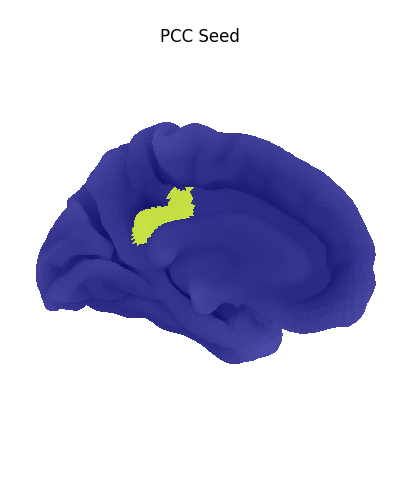```<Figure size 400x400 with 1 Axes>
```

Using a flat mesh can be useful in order to easily locate the area of interest on the cortex. To make this plot easier to read, we use the mesh curvature as a background map.

```bg_map = np.sign(surface.load_surf_data(fsaverage['curv_left']))
# np.sign yields values in [-1, 1]. We rescale the background map
# such that values are in [0.25, 0.75], resulting in a nicer looking plot.
bg_map_rescaled = (bg_map + 1) / 4 + 0.25

plotting.plot_surf_roi(
fsaverage['flat_left'],
roi_map=pcc_map,
hemi='left',
view='dorsal',
bg_map=bg_map_rescaled,
bg_on_data=True,
title='PCC Seed'
)
```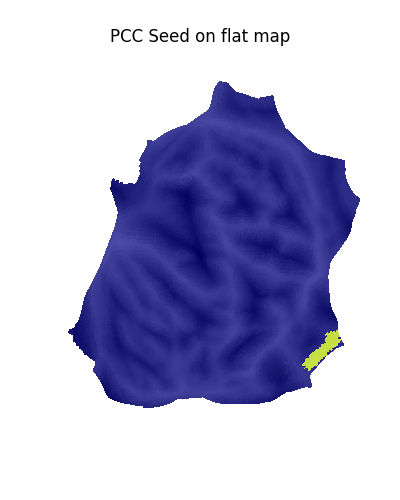```<Figure size 400x400 with 1 Axes>
```

Display unthresholded stat map with a slightly dimmed background

```plotting.plot_surf_stat_map(fsaverage['pial_left'], stat_map=stat_map,
hemi='left', view='medial', colorbar=True,
bg_map=fsaverage['sulc_left'], bg_on_data=True,
darkness=.3, title='Correlation map')
```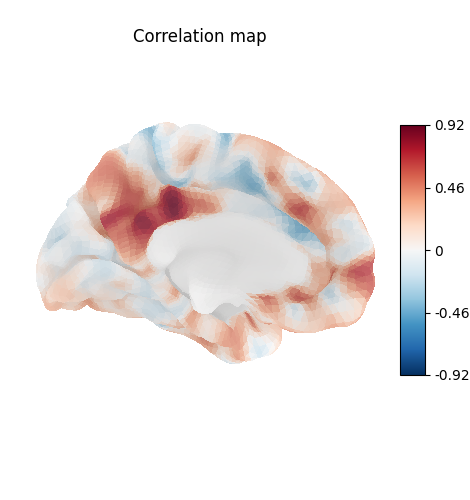```<Figure size 470x400 with 2 Axes>
```

Many different options are available for plotting, for example thresholding, or using custom colormaps

```plotting.plot_surf_stat_map(fsaverage['pial_left'], stat_map=stat_map,
hemi='left', view='medial', colorbar=True,
bg_map=fsaverage['sulc_left'], bg_on_data=True,
cmap='Spectral', threshold=.5,
title='Threshold and colormap')
```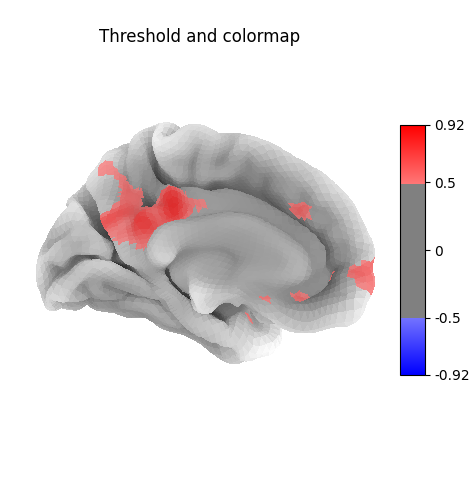```<Figure size 470x400 with 2 Axes>
```

Here the surface is plotted in a lateral view without a background map. To capture 3D structure without depth information, the default is to plot a half transparent surface. Note that you can also control the transparency with a background map using the alpha parameter.

```plotting.plot_surf_stat_map(fsaverage['pial_left'], stat_map=stat_map,
hemi='left', view='lateral', colorbar=True,
cmap='Spectral', threshold=.5,
title='Plotting without background')
```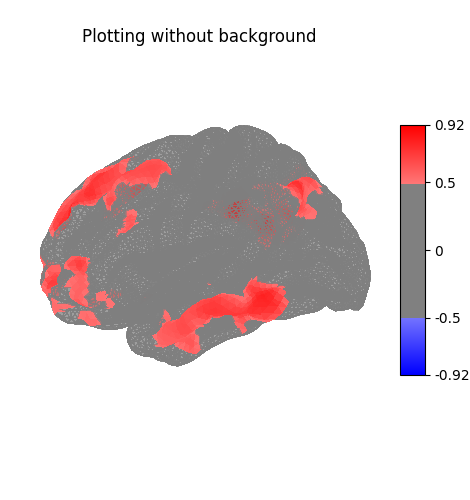```<Figure size 470x400 with 2 Axes>
```

The plots can be saved to file, in which case the display is closed after creating the figure

```plotting.plot_surf_stat_map(fsaverage['infl_left'], stat_map=stat_map,
hemi='left', bg_map=fsaverage['sulc_left'],
bg_on_data=True, threshold=.5, colorbar=True,
output_file='plot_surf_stat_map.png')

plotting.show()
```

Total running time of the script: ( 0 minutes 9.335 seconds)

Estimated memory usage: 9 MB

Gallery generated by Sphinx-Gallery